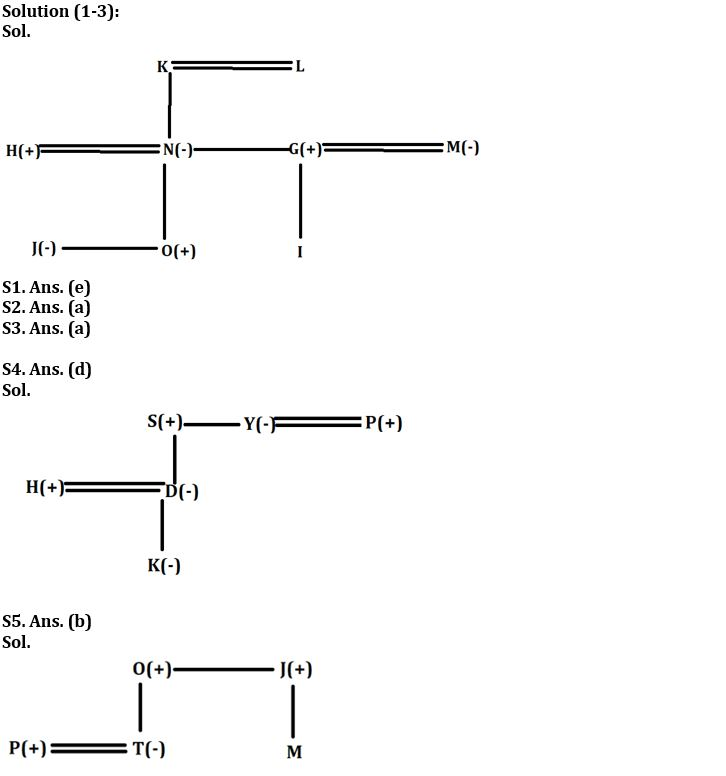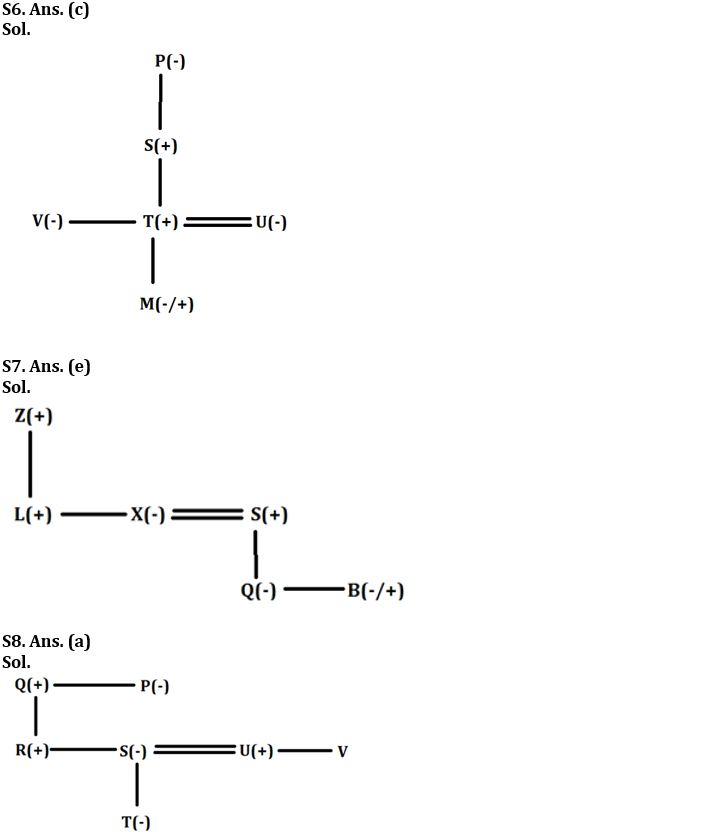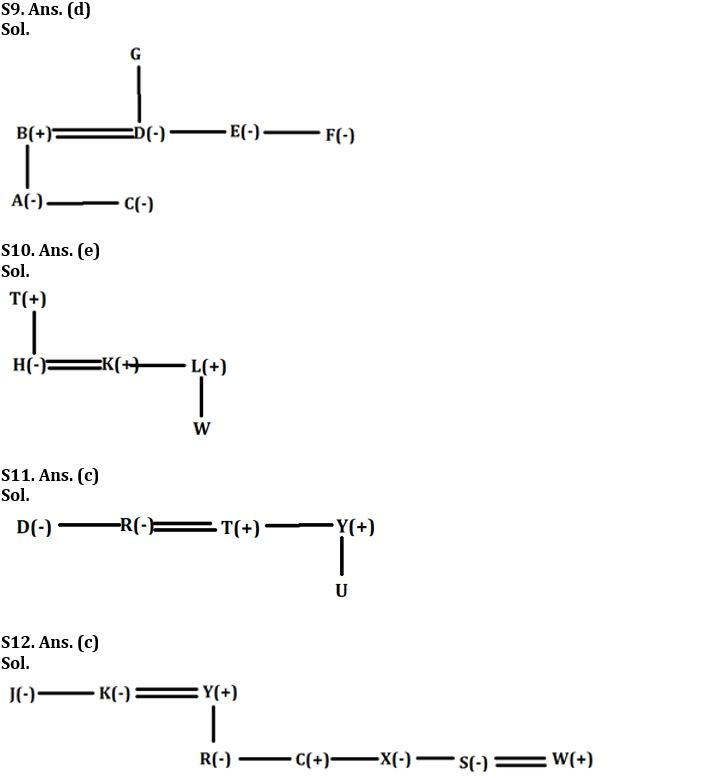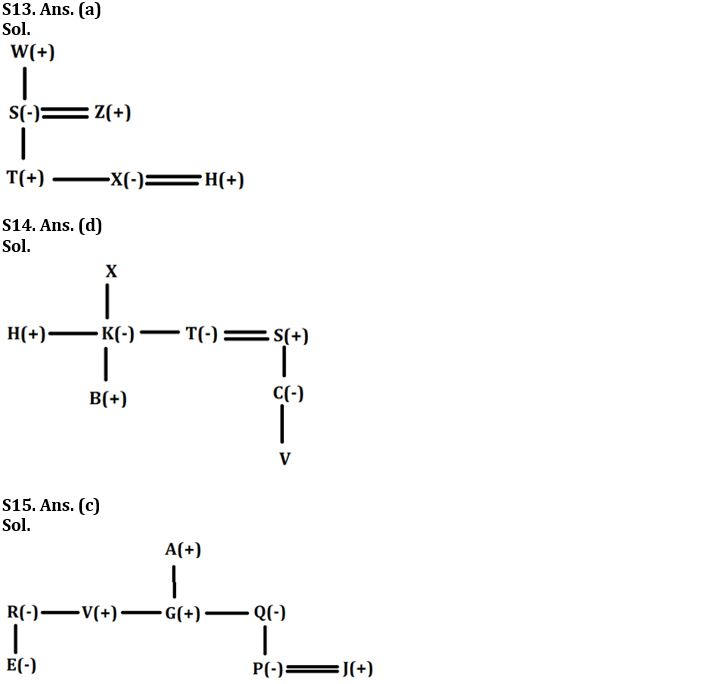Latest Banking jobs   »

# Reasoning Ability Quiz For IBPS RRB PO Clerk Mains 2022- 23rd August

Directions (1-3): Answer the questions based on the information given below.
Nine members G, H, I, J, K, L, M, N and O are members of a family consisting of three generations and three married couples. J is the only sister of O who is the son of N. N is the only daughter of K and L. G is married to the mother of I. Both N and K have only two children. M is the sister-in-law of H and both are married. H is younger to K and older to I.

Q1. Who is the grandfather of I?
(a) K
(b) L
(c) M
(d) H
(e) Can’t be determined

Q2. Which of the following statements is/are correct?
(a) M is the aunt of J
(b) K is the father of G
(c) L is the mother of N
(d) I is the daughter of M
(e) All are correct

Q3. How is J related to L?
(a) Granddaughter
(b) Grandson
(c) Son
(d) Daughter
(e) None of these

Directions (4-5): Answer the questions based on the information given below.
M&N means ‘M is the wife of N
M#N means ‘M is the father of N
M*N means ‘M is the brother of N
M+N means ‘M is the daughter of N
M?N means ‘M is the husband of N

Q4. If ‘H#K+D+S*Y&P’ is true, then how is D related to P?
(a) Mother
(b) Daughter
(c) Sister
(d) Niece
(e) None of the above

Q5. If ‘P?T+O*J#M’ is true then how is M related to O?
(a) Nephew
(b) Cannot be determined
(c) Brother
(d) Son
(e) Brother-in-law

Directions (6-7): Answer the questions based on the information given below.
If X % Y means X is mother of Y,
X\$Y means X is father of Y,
X# Y means X is sister of Y,
X*Y means X is brother of Y,
X+Y means X is wife of Y,
X@Y means X is husband of Y

Q6. If ‘P%S\$V#T@U%M’ is true then how is V related to M?
(a) Father
(b) Brother
(c) Aunt
(d) Grandfather
(e) Grandmother

Q7. If Z\$L*X+S\$Q#B’ is true then how is B related to S?
(a) Father
(b) Sister
(c) Daughter
(d) Son
(e) Cannot be determined

Directions (8-9): Answer the questions based on the information given below.
If, P@Q means P is the mother of Q,
P% Q means P is the sister of Q,
P\$Q means P is the father of Q,
P #Q means P is the brother of Q,
P&Q means P is the daughter of Q.

Q8. In expression ‘P%Q\$R#S@T&U #V’ how is R related to T?
(a) Uncle
(b) Aunt
(c) Mother
(d) Father
(e) None of the above

Q9. In expression ‘A&B\$C&D%E%F&G’ how is G related to A?
(a) Paternal Grandfather
(b) Maternal Grandfather
(c) Maternal Grandmother
(d) Either (b) or (c)
(e) None of the above

Directions (10-12): Answer the questions based on the information given below.
S × T means S is the wife of T,
S – T means S is the brother of T,
S + T means S is the sister of T,
S ÷ T means S is the father of T.

Q10. How is W related to H in the expression ‘T÷H×K–L÷W’?
(a) Son
(b) Nephew
(c) Niece
(d) Son- in -law
(e) None of the above

Q11. Which of the following statement is true if the expression is ‘D+R×T–Y÷U’ true?
(a) U is niece of T.
(b) D is brother-in-law of T
(c) R is sister-in-law of Y
(d) T is father of U
(e) All are correct

Q12. How many female members are in the family if the expression ‘J+K×Y÷R+C–X+S×W’ is true?
(a) One
(b) Two
(c) Five
(d) Four
(e) Can’t be determined

Directions (13-15): Answer the questions based on the information given below.
D @ E – D is the mother of E
D & E – D is the sister of E
D % E – D is the father of E
D # E – D is the brother of E
D ¥ E – D is the wife of E
D £ E – D is the son of E
D ! E – D is daughter of E

Q13. If W % S @ T £ Z % X ¥ H, then how X is related to W?
(a) Granddaughter
(b) Daughter
(c) Son
(d) Sister-in-law
(e) None of the above

Q14. If H#K&T¥S%C@V, B£K!X then who among the following is son-in-law of X?
(a) B
(b) C
(c) H
(d) S
(e) None of the above

Q15. If E!R&V#G£A%Q@P¥J, then which of the following statement is not true?
(a) V is uncle of P.
(b) R is daughter of A
(c) E is nephew of G.
(d) J is son-in-law of Q.
(e) Q is sister of V.

Solutions#### Congratulations!Download Hindu Review of October 2021: Free PDF